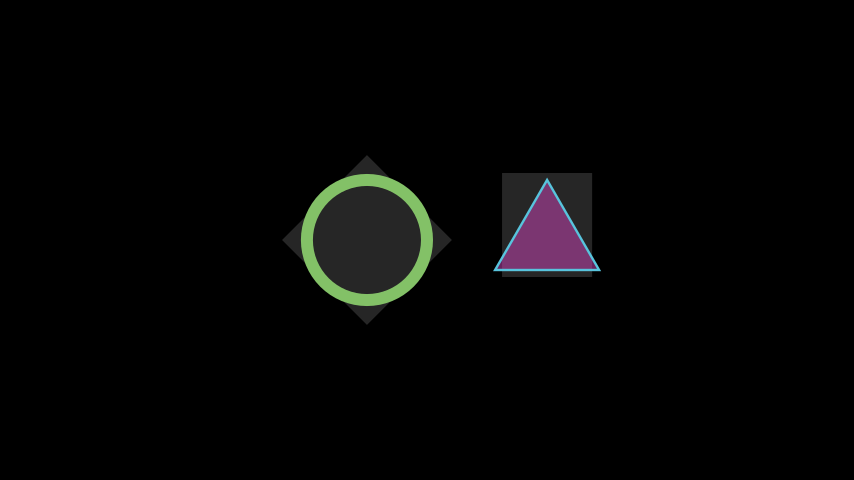# BackgroundRectangle#

Qualified name: `manim.mobject.geometry.shape\_matchers.BackgroundRectangle`

class BackgroundRectangle(mobject, color=None, stroke_width=0, stroke_opacity=0, fill_opacity=0.75, buff=0, **kwargs)[source]#

A background rectangle. Its default color is the background color of the scene.

Examples

Example: ExampleBackgroundRectangle```from manim import *

class ExampleBackgroundRectangle(Scene):
def construct(self):
circle = Circle().shift(LEFT)
circle.set_stroke(color=GREEN, width=20)
triangle = Triangle().shift(2 * RIGHT)
triangle.set_fill(PINK, opacity=0.5)
backgroundRectangle1 = BackgroundRectangle(circle, color=WHITE, fill_opacity=0.15)
backgroundRectangle2 = BackgroundRectangle(triangle, color=WHITE, fill_opacity=0.15)
self.play(Rotate(backgroundRectangle1, PI / 4))
self.play(Rotate(backgroundRectangle2, PI / 2))
```

Methods

 `get_fill_color` If there are multiple colors (for gradient) this returns the first one `pointwise_become_partial` Given two bounds a and b, transforms the points of the self vmobject into the points of the vmobject passed as parameter with respect to the bounds. `set_style`

Attributes

 `animate` Used to animate the application of any method of `self`. `animation_overrides` `color` `depth` The depth of the mobject. `fill_color` If there are multiple colors (for gradient) this returns the first one `height` The height of the mobject. `n_points_per_curve` `sheen_factor` `stroke_color` `width` The width of the mobject.
Parameters
• color (Colors | None) –

• stroke_width (float) –

• stroke_opacity (float) –

• fill_opacity (float) –

• buff (float) –

get_fill_color()[source]#

If there are multiple colors (for gradient) this returns the first one

pointwise_become_partial(mobject, a, b)[source]#

Given two bounds a and b, transforms the points of the self vmobject into the points of the vmobject passed as parameter with respect to the bounds. Points here stand for control points of the bezier curves (anchors and handles)

Parameters
• vmobject – The vmobject that will serve as a model.

• a – upper-bound.

• b – lower-bound

Returns

`self`

Return type

`VMobject`